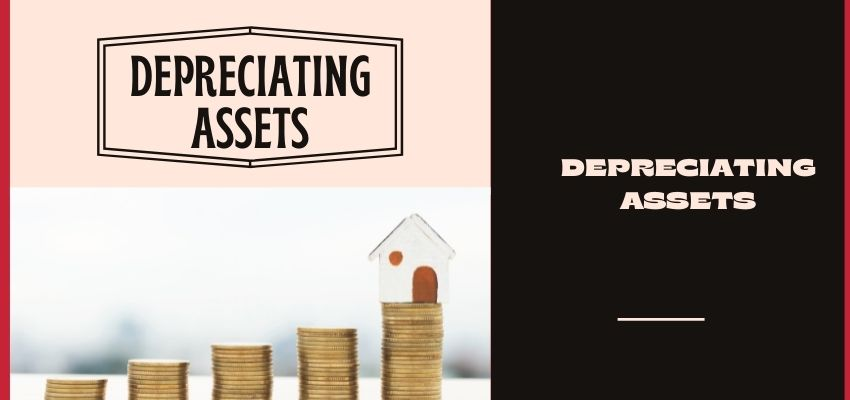# Depreciating Assets

• AccountingDepreciating assets are those business assets that a business can have for a longer period of time to bear future business expenses. These assets are helpful to save us from business taxes, which means the company can get a deduction in tax for the cost of the asset. Like these companies, taxable income is reduced. Every year companies invest some amount of the profit in the depreciating asset. If you want to learn about depreciating assets, then continue reading this blog.

## What is depreciation?

Depreciation is the method where you reduce or save some amount of profit for your business in the form of the asset. This depreciation can be of the short term and for the long term. It depends on the requirement and the selection process of the company. When you buy some depreciate asset then you can manage the flow of money and control your financial status of the company. By writing less money and putting the money in asset a/c.

If you are using different methods and strategies for depreciation then you can decide which asset is useful for life span and which is required for the short term. For example, if you are running ABC company, you might require a laptop, or computer related products then you can depreciate the assets for the 5 years, but you know every year technology change and you know that the computer products after 5 years they will be useless then you can depreciate the product for the 3 years or lesser, then this known as short term depreciation. Every product is having its own depreciating value. Examples of depreciable assets are:

• Buildings
• Computers and software
• Furniture and fixtures
• Land
• Machinery
• Vehicles

### What type of things you can be Depreciating Assets

The Internal Revenu service sets rules and regulations on depreciating assets. Only these types of things can be used for depreciating assets. Also, there are some criteria under with you can do it.

• Need to calculate the usage of the assets. Whether it’s for the long or short term
• Only business earn own it on
• Products should qualify the IRS list.
• You expect it to last more than one year

### What type of information included in the depreciate form

A depreciation form is that form that shows how much assets will be depreciated each year. It also shows how much you have depreciated each and every asset every year. Few things included in the depreciate form:

• What type of asset you are depreciated
• Purchased date of the asset
• Price of the asset which you have paid
• Lifespan period of the asset
• Depreciation method used
• Selling price of the asset, if you sell.

## Types of Depreciating Assets Methods

There are 4 types of depreciating Assetd methods:

• Straight-Line

The straight-line depreciation method is very common and simplest. This method is used to calculating depreciation expenses. In this method, the expense amount is the same every year over the life span of the asset.

Formula:

Depreciation Expense = (AssetCost – Selling value) / Life spam of the asset

• Double Declining Balance

The double-declining-balance depreciation method is a very complicated method to find depreciated assets. This method shows products are more productive when it is purchased and later it is not that much effective. Just like when you buy a car and later year then price of the car is decreased. With the double-declining-balance method, the depreciation factor is 2x more than the straight-line expense method.

Formula

Periodic Depreciation Expense = Beginning book value x Rate of depreciation

• Units of Production

The units-of-production depreciation method is based on the usage of the depreciated assets that means how much time or a number of hours are used or the total number of units to be produced by using the asset, over its life span usage.

Formula:

Depreciation Expense = (Number of units produced / Life in a number of units) x (Cost – selling value)

• Sum-of-the-Years-Digits

The sum-of-the-years-digits method is one of the quickest methods for depreciation. With this, we get higher expenses in the purchased years and a lower expense in the later years of the assets.

Formula

Depreciation Expense = (Remaining life / Sum of the year’s digits) x (Cost – Selling value)